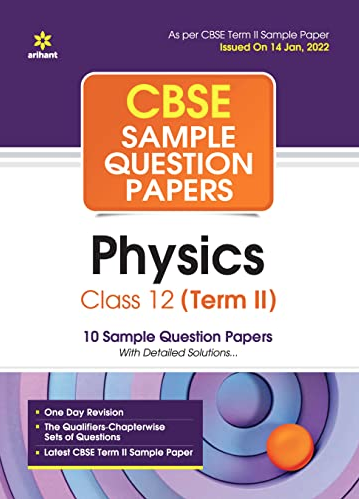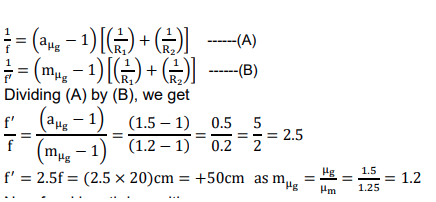# The focal length of a convex lens made of glass of refractive index (1.5) is 20 cm. What will be its new focal length when placed in a medium of refractive index 1.25 ? Is focal length positive or negative? What does it signify?

CBSE Sample Question Paper, Class 12 Physics Term 2 Question - The focal length of a convex lens made of glass of refractive index (1.5) is 20 cm. What will be its new focal length when placed in a medium of refractive index 1.25 ? Is focal length positive or negative? What does it signify?## The focal length of a convex lens made of glass of refractive index (1.5) is 20 cm. What will be its new focal length when placed in a medium of refractive index 1.25 ? Is focal length positive or negative? What does it signify?

### ANS 10 Given aஜౝ = 1.5 Focal length of the given convex lens when it is placed in air is f = + 20 cm Refractive index of the given medium with respect to air is aஜౣ = 1.25 New focal length of the given convex lens when placed in a medium is f ᇱ